# Recap (Spring 2018)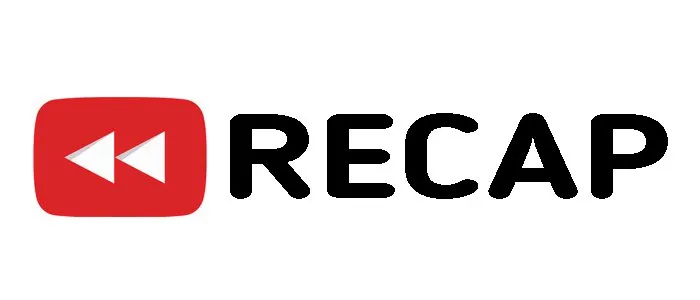Source

## Introduction

Hello its a me again drifter1!

After the previous recap of Winter 2017, we are now getting to a point again where you can't find what you want! I upload so much about many different topics of computer science and information technology, like programming, logic design, networking, mathematics, physics. I write my own compiler during the Compiler Series and even more is yet to come...

If you are interested in only one of these topics than you have problems finding what you want, cause a lot is already been uploaded with a total of 321 posts!

This post will contain every single post uploaded 'till today on this blog in an ordered way and with a small description for each category and each post!

This kind of posts take up a lot of time, effort and patience, and I hope that you appreciate it!

So, without further do, let's get straight into it!

# PROGRAMMING: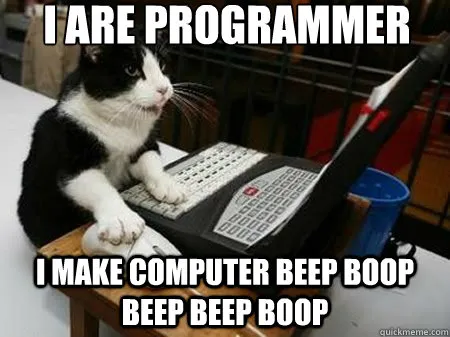Source

Let's start out with my first Category called Programming and split it with the programming languages!

## C:

Basics

C Beginner Codes -> I got into the Basics of C Programming on 2 Example Codes

C Beginner Arrays -> In this post we extended the previous Codes using Arrays and also random

C Pointers, String and Files -> We talked about Pointers, Strings, File and Functions!

C Dynamic Memory Allocation -> I talked off malloc/realloc and used it on an Example Code

C Structs and Switch Case -> I created a switch case menu that handles a struct

Datastructures

C Recursive Algorithms -> We got into Recursions as an reminder for the next posts

C Linked Lists -> We talked about the Linked List Implementation in C

C Binary Trees -> We got into Simple Binary Trees in C

C Queues using Arrays -> Implemetation of a Queue using Arrays

C Stacks using Arrays -> Implementation of a Stack using Arrays

C Queues using Linked Lists -> Implementation of a Queue using a List

C Stacks using Linked Lists -> Implementation of a Stack using a List

C Advanced Lists and Queues -> Double, Circular Linked Lists and Priority Queues

C Advanced Trees -> AVL (implemented), Red-Black (featured) and 2-3 Trees (featured)

C Stack-Queue Exercise using Dynamic Arrays -> Exercise for Stacks-Queues with Arrays

C Stack-Queue Exercise using Linked Lists -> Edited Exercise, but using Lists

C Hashtables with Chaining -> Hashtable Theory and Implementation with Chaining

C Hashtables with Linear Probing -> Implementation with Linear Probing

Other

Can I run a Dual Monitor Setup? (C Code) -> Calculate Angle using Law of Cosines

C Function Comparison -> Compare Functions using Time or Iterations

## Java:

Basics

From C To Java -> I talked off Objects/Classes (introduction), Scanner Input, Printing and Arrays

Java Classes and Methods -> We got into Constructors, Getters-Setters, ToString() and User Defined Methods

Java Composition and ArrayList -> We talked about Java Composition and ArrayLists

Java Inheritance ->  We talked about Inheritance (extends), Abstract Classes and super.toString()

Java Interfaces ->  We talked about Interfaces (implements) and Abstract Methods

Java Exceptions -> We got into Exceptions using the try-catch statement

Java Files -> We got into Textfiles and Binary Files using serializable

Java All-In-One Exercise -> University Task edited to contain only Basics

Java All-In-One Exercise (Solution) -> Solution to the Task

Data Structures

Java Data Structures -> Implementing Lists, Stacks and Queues in Java

Java Graphs Introduction -> Graph Theory and Implementation

Java Graph Traversal Algorithms -> BFS and DFS Traversal Algorithms

Java Graph Minimum Spanning Tree Algorithms -> Prim and Kruskal MST Algorithms

Java Graph Shortest Path Algorithm (Dijkstra) -> Dijkstra Algorithm

Java Graph Shortest Path Algorithm (Bellman-Ford) -> Bellman-Ford Algorithm

Java Graph All Pair Shortest Path Algorithms (Floyd-Warshall/Johnson) -> Floyd-Warshall and Johnson Algorithms

Java Graph Maximum Flow Algorithm (Ford-Fulkerson) -> Ford-Fulkerson Algorithm

Java Graph (Backtracking) Hamiltonian Circuit Algorithm -> Backtracking Hamiltonian Cycle Algorithm

Java Graph Eulerian Circuit Detection Algorithm -> Eulerian Cycle Detection Algorithm

Java Graph Minimum Spanning Tree Algorithms 2 -> Boruvka and Reverse Delete Algorithms

More Graph Algorithms are yet to come...

GUI

Java GUI (awt) -> Getting into GUI Programming using the awt library

Java GUI (swing) -> Extend previous Knowledge using the swing Library and JOptionPane's

Java GUI Events and Listeners -> Getting into Events and ActionListeners

Java GUI Examples -> Examples of GUI's that implement everything told

Java All-In-One Exercise Extended -> Previous Task Edited to include GUI

Java All-In-One Exercise Extended (Solution) -> Solution to the Task

Java Web Applets -> Writing a Applet in Java

## Assembly:

Basics

Assembly Basic Coding Structure -> Introduction to Assembly

Assembly Input/Output Calls -> System Calls to Input and Output

Assembly Branches and Jumps -> Getting into Branches, Jumps that help us write if, for, while statementsAssembly Arrays and Memory Instructions -> Arrays and sw, lw instructions

Assembly Code Examples -> Examples to make sure everything until now is understandable

Assembly Functions and Pseudodynamic Arrays -> Switch case that calls functions for an pseudodynamic Array

Assembly Stack and Recursive Algorithms -> Stack Introduction and 3 Recursion Code Examples

Assemly Heap Memory Allocation -> Heap Allocation and Dynamic Memory

Assembly File Inputs/Output Calls -> File Input and Output using System Calls

Assembly Stack Datastructure -> Stack Implementation in Assembly

Assembly Floating Point Register Example (Trading Profit Calculator) -> Floating Point Register

# LOGIC DESIGN: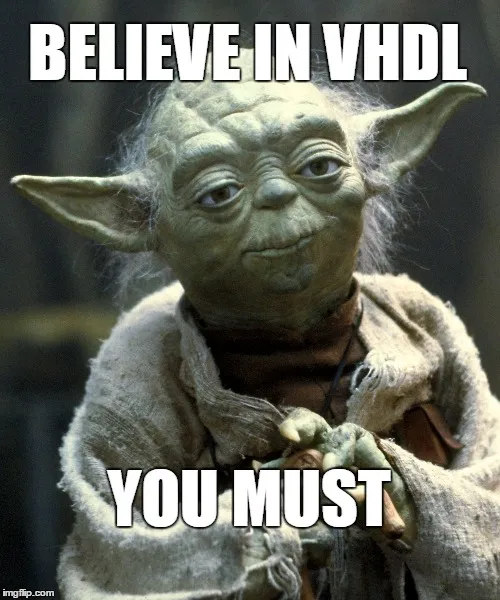Source

Theory

Numeral Systems -> Numeral Systems, Conversions, Operations

Logical (Boolean) Functions -> Boolean Expressions, Truth Tables, Minterms, Maxterms

Boolean Algebra and Simplification Theorems -> Boolean Algebra, Laws and De Morgan

Karnaugh Map Simplification -> Karnaugh Maps

Basic Logic Gates -> NOT, OR, AND, XOR and XNORGates

Universal Logic Gates -> NOR and NAND Logic

From Function to Circuits using Multi-Input Gates -> Multi-Inputs Gates and Tips

Adder Circuits -> Half, Full and N-bit Adder Circuits

Multiplexer, Encoder and Decoder Circuits -> MUX, Encoder and Decoder Circuits

Waveforms and Clocks -> Waveforms and Clocks, How to test all Inputs

Latches and Flip Flops -> Latches and D, T, JK Flip Flops

Sequential (Synchronous) Circuits -> State Table, Equations and Diagram

Quine McCluskey (Tabular) Simplification Method -> Example Tabular Simplication

Statetable Simplification and Implementation with one-hot encoding -> Staircase DFF Implementation

Binary Decision Diagrams -> Binary Decision Trees, Diagrams and ROBDD's

Multisim Implementation

Multisim Getting Started Tutorial -> Multisim Introduction

Logic Gates in Multisim -> Testing out Logic Gates in Multisim

Simple Circuits in Multisim -> Circuits using Logic Gates in Multisim in 3 ways

Adder Circuits in Multisim -> Implementing Half, Full and N-bit Adders in Multisim

Multiplexer, Encoder and Decoder Circuits in Multisim -> Multisim Implementation Examples

Advanced Sequential Circuits in Multisim -> JK Flip Flop Implementation of a State Diagram

VHDL

VHDL Introduction -> What are HDL Languages, VHDL Theory

VHDL Basic Coding Structure -> Structure, Libraries, Assignment Statements and Processes

VHDL Basic Circuits -> Simple VHDL Coding using knowledge from the previous post

VHDL Modelsim Getting Started Tutorial -> Modelsim Tutorial

VHDL Behavioral, Dataflow and Structural Models -> Coding Models

VHDL Sequential Circuits -> Reminders, D-Flip-Flops, Registers and Counters

VHDL Testbench and Datatypes -> Testbench, Constants, Variables and Datatypes

VHDL Finite-State Machines -> FSM Coding

VHDL Coffee FSM Example -> Coffee FSM

Simple ALU Circuit Project in 3 parts...

VHDL Simple ALU Circuit(part 1) -> ALU Layout/Operations, Adder, Subber and Incrementation/Decrementation Components

VHDL Simple ALU Circuit(part 2) -> Multiplicator and Comparator Component

VHDL Simple ALU Circuit(part 3) -> Logical Operation (NOT, AND, OR, XOR), Structuring/Combining and Simulation/Testing

Simple FPGA Design using Quartus in VHDL -> Simple FPGA Design

This category had no new posts for 4 months now...This will change soon :)

# NETWORKING:Source

In this category are programming posts in C and Java that have to do with Inter-process or Inter-network communication. I use Linux for the C posts and get into everything you need to get started with Socket programming. In Java we simply get into how the Java Networking API works in some simple examples. I also started with Distributed algorithms using MPI that we will continue for sure!

C

C Processes in Linux -> fork() mainly

C Threads in Linux -> pthreads library

C Process/Thread Synchronization in Linux -> Critical Section, Sync. Algorithms, Semaphores

C Inter-Process Communication in Linux -> Shared Memory, Pipes and Message Queues

C Signals and Signal Handling in Linux -> Signals, Signal Handling

C Classic Synchronization Problems in Linux -> Producer-Consumer, Readers-Writers, Dining Philosophers

C Sockets in Linux -> TCP and UDP Sockets, Libraries and Datatypes

C Server-Client Communication in Linux -> Server-Client Implementation/Communication

C Server-Client Kinds in Linux -> Kinds of Clients/Servers, Full-On Example

Java

Java Threads and Synchronization -> Java Threads, synchronized

Java Sockets and Server-Client Communication -> Sockets, server-client programm

MPI

Distributed algorithm for Routing (Toueg) -> an introduction to distribution, MPI and also a implementation of Toueg's Routing algorithm using MPI

We will do more algorithms and I'm also thinking of getting into some Networking Theory!

# MATHEMATICS:
Source

This category is all about maths...I will get into all the basic and more advanced concepts of many different branches of math. We will also get into how we use it in computer science or information technology, and how useful mathematics actually are! I know that many of you hate and are scared of math, but I will try to make everything seem as simple as possible, so that you only get to know what you actually need. All these advanced mathematics will be needed when we get into Physics or even Game Design!Let's split the posts based on the branch...

Linear Algebra:

Linear Algebra Introduction -> Tables and Linear Systems

Linear Algebra Gauss Method -> Gauss Method

Linear Algebra Inverse Matrix and Determinants -> Gauss-Jordan for finding Inverse Matrix, Determinants

Linear Algebra Vector Spaces -> Vector Spaces

Linear Algebra Combinations and Independence -> Combinations and Independence

Linear Algebra System Examples -> Linear System Examples

Linear Algebra Functions -> Functions

Linear Algebra Function Matrix and Special Cases -> Function Matrix and Special Cases

Linear Algebra Eigenvalues and eigenvectors -> Eigenvalues, Eigenvectors

Linear Algebra Function Examples -> linear function examples

Mathematical Analysis:

Single-Variable

Mathematical Analysis Introduction (Functions) -> Functions, Operations, Basics

Mathematical Analysis Functions II -> Monotony, Extremum, Categories

Mathematical Analysis Limits and Continuity -> Limits, Continuity

Mathematical Analysis Continuity II -> more stuff about Continuity

Mathematical Analysis Limit Solving Examples (with roots) -> Limit examples with roots mainly

Mathematical Analysis Derivatives -> Derivatives, Rules, common functions

Mathematical Analysis Differentiation Theorems -> Fermat, Rolle, Mean value, L'Hospital, ...

Mathematical Analysis Indefinite and Riemann Integrals -> Indefinite-definite Integral Properties

Mathematical Analysis Integration by Substitution Technique -> Substitution Technique

Mathematical Analysis Integration by Parts Technique -> by Part Integration

Mathematical Analysis Integration Techniques for Rational Functions -> Rational function integration

Mathematical Analysis Integration Techniques for Trigonometric Functions -> Trigonometric function integration

Mathematical Analysis Integral Applications and Examples -> Area, Volume, Length of Curve

Mathematical Analysis Sequence Basics -> Sequence, bounding, monotony

Mathematical Analysis Subsequences and Convergence -> Subsequences and Convergence

Mathematical Analysis Special Sequences and Divergence -> Special Sequences and Divergence

Mathematical Analysis Series Basics -> Series basics

Mathematical Analysis Series Convergence Tests -> Series convergence

Mathematical Analysis Series Convergence Examples -> examples using the tests of the previous post

Mathematical Analysis Power Series -> Power Series generally

Mathematical Analysis Taylor and Maclaurin Series -> Taylor and Mclaurin Power Series

Mathematical Analysis Fourier Series -> Fourier series

Multi-Variable

Mathematical Analysis Vectors, Lines and Planes -> what the title says

Mathematical Analysis Advanced Plane Types -> Plane Types

Mathematical Analysis Multivariable and Vector Functions -> Multi-Val and Vector Functions

Mathematical Analysis Partial Derivatives -> Partial derivatives

Mathematical Analysis Directional Derivatives -> Directional derivatives

Mathematical Analysis Total Differential -> Total differential and does f exist with such df

Mathematical Analysis Double and Multiple Integrals -> double and multiple integrals

and some more posts are yet to come :)

Differential equations:

Differential equations Introduction -> definition, applications

Ordinary first-order Differential equations (part 1) -> types, separable, homogeneous, exact

Ordinary first-order Differential equations (part 2) -> linear n-order, linear, Bernoulli and Riccati first-order

First-order Differential equation exercises -> exercises

Linear second-order Differential equations with constant coefficients -> linear second-order with const coeffs

Euler, Wronsky and Canonical linear second-order Differential equations -> euler, wronsky, lagrange

Second-order Differential equation exercises -> exercises

Laplace method for solving Differential equations -> laplace method

Collabs:

Collaborative posts that fit to more then one category...

Linear Algebra Method for solving Differential Equations -> table method for solving simple ODE's

In these gigantic posts I explain everything about some topic of Maths!

All about Complex numbers -> complex number theory in depth

And more is yet to come!!!

# PHYSICS: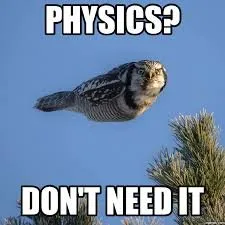Source

In this category we get into different Branches of Physics. For each branch there will be a lot of theory explained in an very analytic way and even with posts that contain only examples/exercises for the different concepts, theorems and so on...This means that you will understand everything easily and will also know how to apply it on very descriptive problems!

Intro

Physics Introduction -> what is physics?, Models, Measuring

Vector Math and Operations -> Vector mathematics and operations (actually mathematical analysis, but I don't got into that before-hand :P)

Classical Mechanics

Velocity and acceleration in a rectlinear motion -> velocity, accelaration and averages of those

Rectlinear motion with constant accelaration and free falling -> const accelaration motion and free fall

Rectlinear motion with variable acceleration and velocity relativity -> integrations to calculate pos and velocity, relative velocity

Rectlinear motion exercises -> examples and tasks in rectlinear motion

Position, velocity and acceleration vectors in a plane motion -> position, velocity and accelaration in plane motion

Projectile motion as a plane motion -> missile/bullet motion as a plane motion

Smooth Circular motion -> smooth circular motion theory

Plane motion exercises -> examples and tasks in plane motions

Force and Newton's first law -> force, 1st law

Mass and Newton's second law -> mass, 2nd law

Newton's 3rd law and mass vs weight -> mass vs weight, 3rd law, friction

Applying Newton's Laws -> free-body diagram, point equilibrium and 2nd law applications

Contact forces and friction -> contact force, friction

Dynamics of Circular motion -> circular motion dynamics, applications

Object equilibrium and 2nd law application examples -> examples of object equilibrium and 2nd law applications

Contact force and friction examples -> exercises in force and friction

Circular dynamic and vertical circle motion examples -> exercises in circular dynamics

Advanced Newton law examples -> advanced (more difficult) exercises

For now we only talked about Classical Mechanics (that is also unfinished), but there will also be: Electromagnetism, Thermodynamics, Relativity, Quantum Mechanics, Optical Physics, Atomic and Molecular Physics, Particle Physics, Nuclear Physics, Astrophysics :)

# Writing a simple Compiler on my own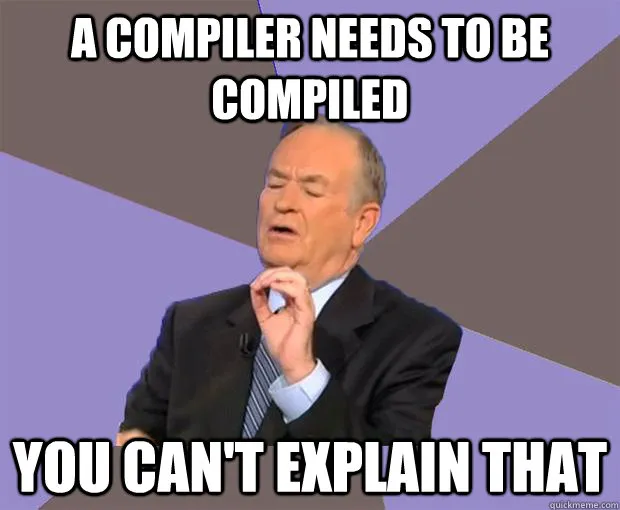Source

This is a series where I write my own compiler for a simple C-like language using the C-tools Flex and Bison and explaining any compiler theory needed in between! This series takes up a lot of time and so posts are very rare :P

Introduction -> What is a compiler, what you have to know and what you will learn

A simple C Language -> Simplified C, comparison with C, tokens, basic structure

Lexical Analysis using Flex -> Theory, Regular Expressions, Flex, Lexer

Symbol Table (basic structure) ->Why Symbol Tables, Basic Implementation

Using Symbol Table in the Lexer -> Flex and Symbol Table combination

Syntax Analysis Theory -> Syntax Analysis, grammar types and parsing

Bison basics -> Bison tutorial actually

Creating a grammar for our Language -> Grammar and first Parser

Combine Flex and Bison -> lexer and parser combined

The posts that will follow will have to do with:

• passing information from the lexer to the parser (and fixing some stuff)
• finishing of the grammar/parser (more rules)
• Semantic analysis (predicates, more about the symbol table)
• Abstract syntax tree (intermediate code)
• Machine code generation (MIPS Assembly)

After that we can also do Optimizations, Extensions and more if you like :)

# OTHER:Source

I also post random stuff sometimes...

For example the posts following talk about my opinions on Cryptocurrency :)

My Opinions on the whole Crypto Bear Market -> My history with crypto, what I trade, the bear market, steem

STEEM up 45% - What's next? -> state of the cryptosphere, questions you should ask yourself, advices

And those are not all categories yet! I also want to get into Databases, Game Design (Unity), Artificial Intelligence (AI) and even more...in the near future :P

Also, what do you thing of the idea of Live-Streaming in DLive? :)

And this is actually it and I hope that you liked the introduction of memes in between!

I also hope that this post will help you while searching for specific content, topics and stuff on my blog, cause with so many different posts and such a complicated uploading order everything seems so messed up!

Bye!

H2
H3
H4
3 columns
2 columns
1 column